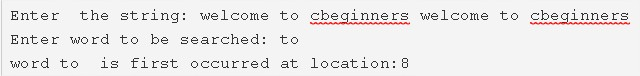# C Program To Find First Occurrence Of A Word In String | C Programs

C program to find the first occurrence of a word in a given string – In this article, we will detail in on the ways to find the first occurrence of a word in a given string in C programming.

Suitable examples and sample programs have also been added so that you can understand the whole thing very clearly. The compiler has also been added with which you can execute it yourself.

The ways used in this distinct post are as follows:

• Using Standard Method
• Using Function

A string is nothing but an array of characters. The value of a string is determined by the terminating character. Its value is considered to be 0.As given in the example above, firstly, you need to enter the string that you want to investigate.

The string entered, in this case, is as follows:

“welcome to cbeginners welcome to cbeginners”

Then, enter the word that you want to search for.

The word “to” is chosen in this situation.

Hence, it is seen that the word “to” occurs for the first time at location 8.

Thus, the multiple methods to do the same in C programming are as follows:

## Using Standard Method

1. Read the entered string and store the string in the variable ‘s’, using gets(s) function.

2) Read the word to be searched and store the word in the variable ‘w’ using gets(w) function.

3) i=0, Iterate the while loop until the last character of the string becomes to null.

a) If s[i] of the string  is matched with the first letter of the word then

Initialize k=1.

The for loop iterates through the word with the structure for(c=1;w[c]!=’\0′;c++), compare w[c] with the next element s[i+c] of the string. If matches then go for next comparison by increasing c value. If not matched then initialize k=0 and terminate the “for loop”.

b) If k=1 then initialize index=i.

c) Increase the i value to go through the next element of the string and initialize k=0(go to step 3).

4) If k=1 then print the index value which is the first occurrence of the word in the string. Otherwise, print the word “does not occur” in the string.

Output:

### Using Function

1. The main() calls the check() function,  passing the string and word as arguments to the check() function. The check() function finds the first occurrence of the word in the string.

2) Using 1st for loop store the index values of the white spaces present in the string, into the integer string a[].

3)The 2nd for loop iterates through the white spaces of the string with the structure for(i=0; i<k;i++)

a) If the index of the 1st white space is equal to the length of the word then initialize t=0 and

compare the 1st character of the word w[l] with the first element of the string s[l+j]if both are matched then increase the t value. Repeat this step by increasing l value until the last character of the word.

b) If t=length of the word then found=1 and break the loop.

If found=1 then the function returns the j value otherwise return -1.

4) j value indicates the first occurrence of the word in the string.

Output:

x

## C Program To Check If Alphabet, Digit or Special Character | C Programs

C program to check for an alphabet, digit or special character – In this article, ...